# Law of Conservation of Energy

It states that, enegy can neither be created nor be destroyed. The energy of an isolated system is constant.

∆U = q + W

where, ∆U = internal energy change

q = heat added to system

W = work added to system

Sign convention

• q is +ve = heat is supplied to the system
• q is -ve = heat is lost by the system
• W is +ve = work done on the system
• W is -ve = work done by the system

# Work (Pressure-volume Work)

Let us consider a cylinder which contains one mole of an ideal gas in which a frictionless piston is fitted.

Total volume of the gas = V

Pressure of the gas = P

External pressure = Pex

If

Pex > P

Final volume = Vf

Distance moved by the piston = l

Cross-sectional area of piston = A

Volume change ∆V = l x A = (Vf – Vi)

P= F/A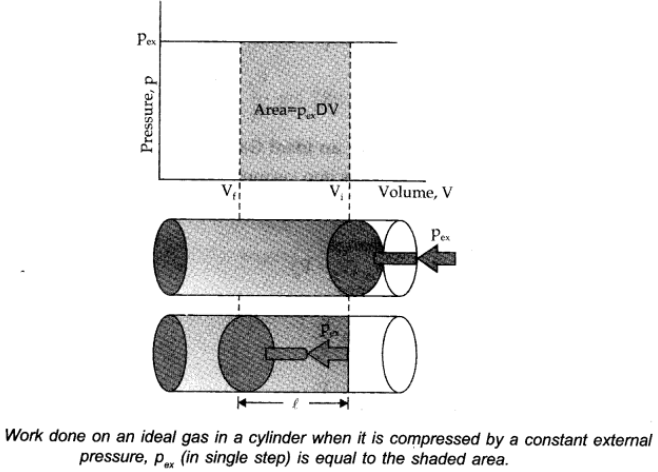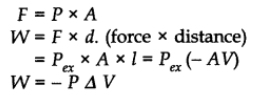-ve sign is used for work done by the system in case of expansion in volume by conventions.

If in a system volume expands from V1 to V2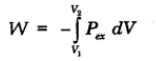## Work Done in Isothermal and Reversible Expansion of Ideal Gas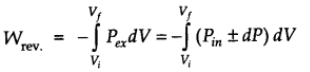Since dP x dV is very small we can write,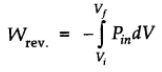For ideal gas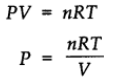Therefore, at constant temperature,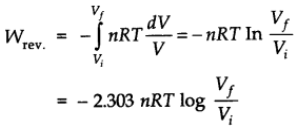## Isothermal and Free Expansion of an Ideal Gas

For isothermal expansion of an ideal gas into vacuum W = 0

Since, Pex = 0

∆U = 0, q = 0

• For isothermal irreversible change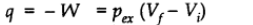• For isothermal reversible change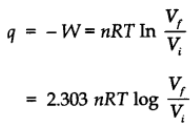For adiabatic change q = 0

# Thermodynamic Process

It is the operation which brings change in the state of the system.

Thermodynamic processes are:

1. Isothermal process: In which temperature remains constant, i.e., (dT = 0, ∆U = 0).
2. Adiabatic Process: It is a process in which no transfer of heat between system and surrounding, takes place. i.e., (∆q = 0).
3. Isobaric process: When the process is carried out at constant pressure, it is said to be isobaric. i.e., (dP = 0, ∆P =0).
4. Isochoric process: A process when carried out at constant volume, it is known as isochoric in nature. i.e., (∆V = 0).
5. Cyclic process: It is process in which system returns to its original state after undergoing a serious of change. i.e., (∆Ucyclic = 0; ∆Hcyclic = 0).
6. Reversible process: A process that follows the reversible path, i.e., the process which occurs in infinite number of steps in this way that the equilibrium conditions are maintained at each step, and the process can be reversed by infinitesimal change in the state of function.
7. Irreversible process: The process which cannot be reversed and amount of energy increases. All natural process are Irreversible.